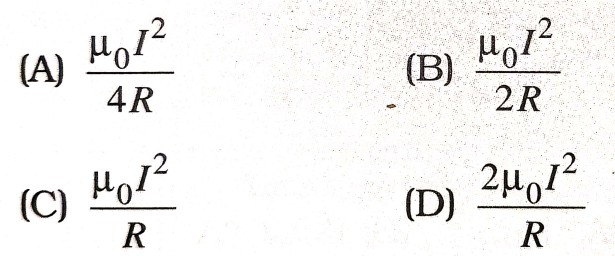MAGNETISM AND MATTER

1. Which of the following has a low value in ferrites ?

(A) Conductivity
(B) Permeability
(C) Magnetic susceptibility
(D) All the above

2. The dimensional representation of magnetic flux density is ;

(A) [MLT-2]
(B) [MLT-2A-1]
(C) [MLT-2A-2]
(D) [MT-2A-1]

3. Tangent law is applicable only when :

(A) two uniform and mutually perpendicular magnetic fields exist
(B) two magnetic fields exist
(c) horizontal component of earth’s magnetic field is present
(D) uniform magnetic field are used

4. Ferrites may be :

(A) ant ferromagnetic
(B) ferromagnetic
(C) ferrimagnetic
(D) None of the above

5. A magnetic dipole moment is a vector quantity directed from :

(A) S to N
(B) N to S
(C) E to W
(D) W to E

6. The force acting per unit length of a semi circular wire of radius R carrying a current I is :7. Which of the following has higher magnetic susceptibility ?

(A) diamagnetic
(B) paramagnetic
(C) ferromagnetic
(D) None of these

8. The magnetic field of earth is due to :

(A) induction effect of the sun
(B) the presence of a large magnet at the centre of the earth
(C) interaction of cosmic rays with the current of earth
(D) motion and distribution of some material in an outside the earth

9. Direction of magnetic field can determined by :

(A) declination only
(B) inclination only
(C) inclination and horizontal component of field
(D) declination and horizontal component of field

10. The dimensions of magnetic permeability are :

(A) [MLT-2A-2]
(B) [ML2T2A-2]
(C) [ML2T-2A-1]
(D) [M-1LT-2A-2]

11. A magnetic field exerts no force on a/an :

(A) magnetic
(B) unmagnetised iron bar
(C) moving charge
(D) charge at rest

12. Susceptibility is +ve and large for substances.

(A) ferromagnetic
(B) paramagnetic
(C) diamagnetic
(D) non-magnetic